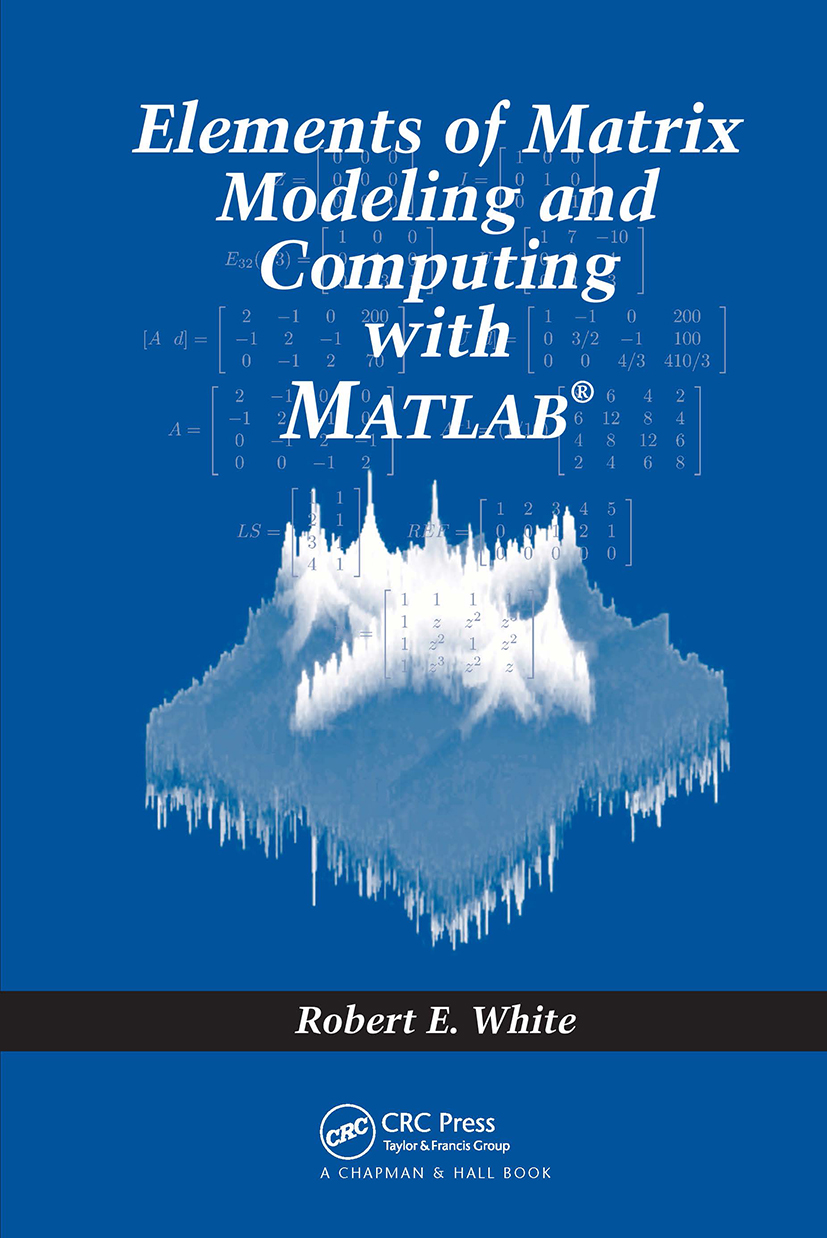# Elements of Matrix Modeling and Computing with MATLAB

## 1st Edition

Chapman and Hall/CRC

402 pages | 116 B/W Illus.

Hardback: 9781584886273
pub: 2006-09-12
SAVE ~\$23.00
\$115.00
\$92.00
x
eBook (VitalSource) : 9780429121098
pub: 2006-09-12
from \$57.50

FREE Standard Shipping!

### Description

As discrete models and computing have become more common, there is a need to study matrix computation and numerical linear algebra. Encompassing a diverse mathematical core, Elements of Matrix Modeling and Computing with MATLAB examines a variety of applications and their modeling processes, showing you how to develop matrix models and solve algebraic systems. Emphasizing practical skills, it creates a bridge from problems with two and three variables to more realistic problems that have additional variables.

Elements of Matrix Modeling and Computing with MATLAB focuses on seven basic applications: circuits, trusses, mixing tanks, heat conduction, data modeling, motion of a mass, and image filters. These applications are developed from very simple to more complex models. To explain the processes, the book explores numerous topics in linear algebra, including complex numbers and functions, matrices, algebraic systems, curve fitting, elements of linear differential equations, transform methods, and tools of computation. For example, the author uses linearly independent vectors and subspaces to explain over- and under-determined systems, eigenvalues and eigenvectors to solve initial value problems, and discrete Fourier transforms to perform image filtering in the frequency domain. Although the primary focus is to cultivate calculation skills by hand, most chapters also include MATLAB to help with more complicated calculations.

List of Figures

List of Tables

Preface

Introduction

VECTORS IN THE PLANE

Floating Point and Complex Numbers

Complex Valued Functions

Vectors in R2

Dot Product and Work

Lines and Curves in R2 and C

VECTORS IN SPACE

Vectors and Dot Product

Cross and Box Products

Lines and Curves in R3

Planes in R3

Extensions to Rn

Ax = d: UNIQUE SOLUTION

Matrix Models

Matrix Products

Special Cases of Ax = d

Row Operations and Gauss Elimination

Inverse Matrices

LU Factorization

Determinants and Cramer's Rule

Ax = d: LEAST SQUARES SOLUTION

Curve Fitting to Data

Normal Equations

Multilinear Data Fitting

Parameter Identification

Ax = d: MULTIPLE SOLUTIONS

Subspaces and Solutions in R3

Row Echelon Form

Nullspaces and Equilibrium Equations

LINEAR INITIAL VALUE PROBLEMS

First Order Linear

Second Order Linear

Homogeneous and Complex Solution

Nonhomogeneous Linear Differential Equations

System Form of Linear Second Order

EIGENVALUES AND DIFFERENTITAL EQUATIONS

Solution of x' = Ax by Elimination

Real Eigenvalues and Eigenvectors

Solution of x' = Ax + f (t)

IMAGE PROCESSING IN THE SPACE DOMAIN

Matrices and Images

Contrast and Histograms

Blurring and Sharpening

IMAGE PROCESSING IN THE FREQUENCY DOMAIN

Laplace and Fourier Transforms

Properties of DFT

DFT in Rn × Rn

Frequency Filters in Rn × Rn

Appendix: Solutions to Odd Exercises

Bibliography

Index